Reconstruction of the 2016 Reigate Hill formation 1.Draw a circle. Draw and extend the horizontal and vertical centerlines. 2.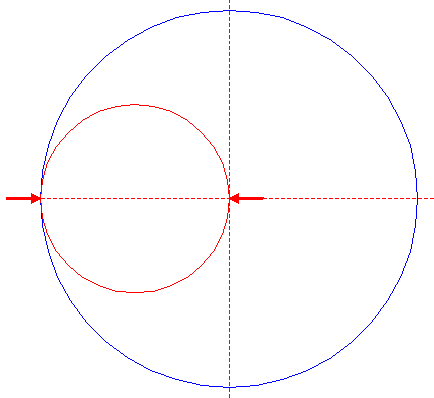Construct a "two-points" circle (defined by the two end-points of a centerline) between the center of circle 1 and its lefthand intersection with the horizontal centerline. 3.Construct a "two-points" circle between the centers of circles 1 and 2. 4.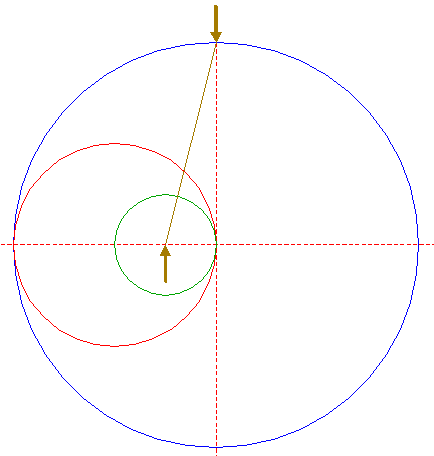Draw the connecting line between the upper intersection of circle 1 and the vertical centerline, and the center of circle 3. 5.Construct the bisector of the acute angle between line 4 and the horizontal centerline. 6.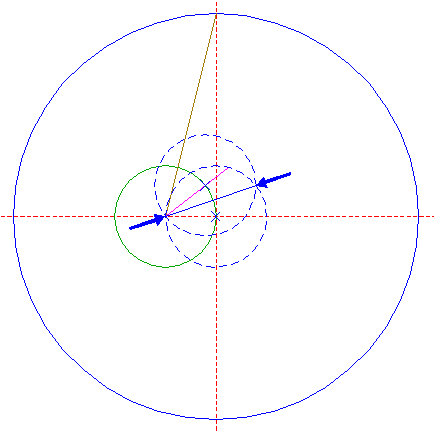Construct the bisector of the acute angle between line 5 and the horizontal centerline. 7.Construct a line through the center of circle 3, perpendicular to line 6, downwards. 8.Construct the bisector of the right angle between lines 6 and 7. 9.Construct a "two-points" circle between the intersection of line 8 and the upper intersection of circle 1, both with the vertical centerline. 10.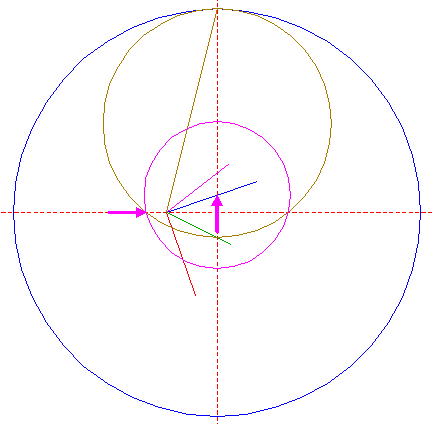Construct a circle centered at the intersection of line 6 and the vertical centerline, passing through the lefthand intersection of circle 9 and the horizontal centerline. 11.Construct a line through the upper intersection of circle 10 with, and perpendicular to the vertical centerline, extending to the left upto circle 1. 12.Construct a circle centered at the upper intersection of circle 1 and the vertical centerline, passing through the intersection of circle 1 and line 11. 13.Copy circle 12 sixteen times, the first to its first intersection counterclockwise with circle 1, then consecutively to the corresponding intersections of the copied circles, in the shown sequence. 14.Draw the connecting lines between all adjacent intersections of circles 12 and 13 with circle 1.Steps 2 - 14 together are the ruler-and-compass (exact) construction of the inscribed heptadecagon (regular 17-sided polygon) of circle 1, pointing up. It is based upon Eric Weisstein's description on Wolfram's MathWorld.Number the intersections (angular points) 1 - 17 clockwise, starting at the top. 15.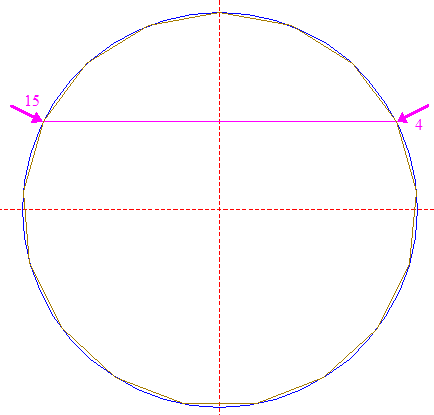Draw diagonal 4-15 (diagonal of heptadecagon 14 between angular points 4 and 15). 16.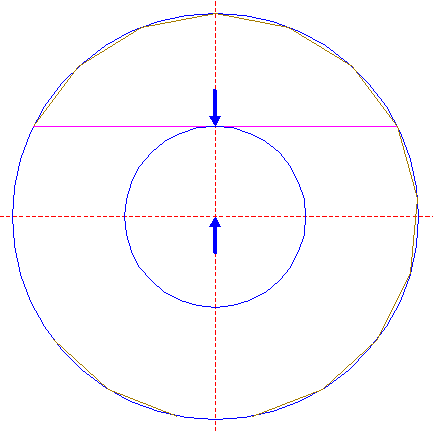Construct a circle concentric to circle 1, tangent to line 15. 17.Draw rays 7 and 12 (from the center of circle 1 to the angular points 7 and 12 of heptadecagon 14). 18.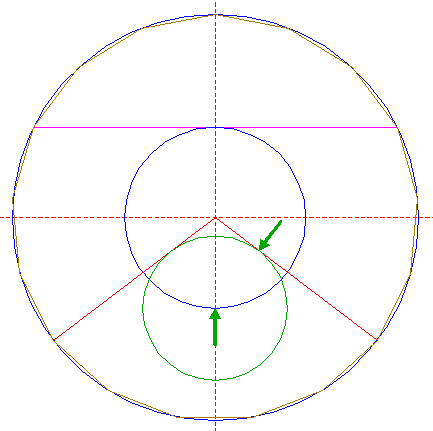Construct a circle centered at the lower intersection of circle 16 and the vertical centerline, tangent to lines 17. 19.Construct a circle concentric to circle 1, passing through the intersections (tangent points) of circle 18 and lines 17. 20.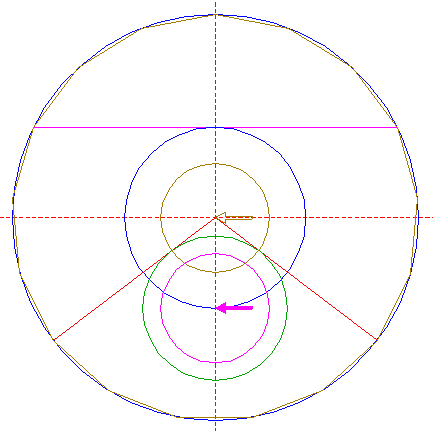Copy circle 19 to the center of circle 18. 21.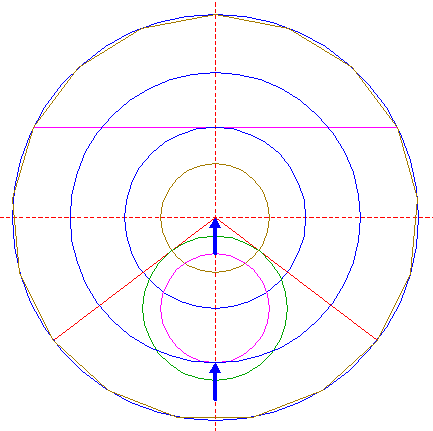Construct a circle concentric to circle 1, tangent to circle 20 at the lower side. 22.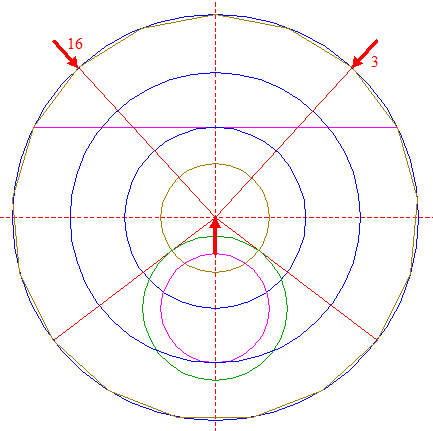Draw rays 3 and 16. 23.Draw the connecting line between the intersections of circle 21 and lines 22. 24.Draw rays 2 and 17. 25.Construct a circle concentric to circle 1, passing through the intersections of lines 23 and 24. 26.Copy circle 18 two times, to the intersections of circle 25 and lines 17. 27.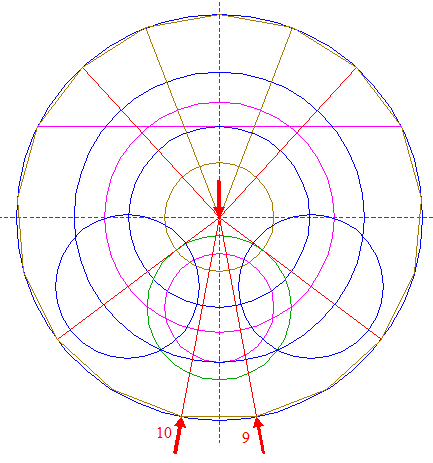Draw rays 9 and 10. 28.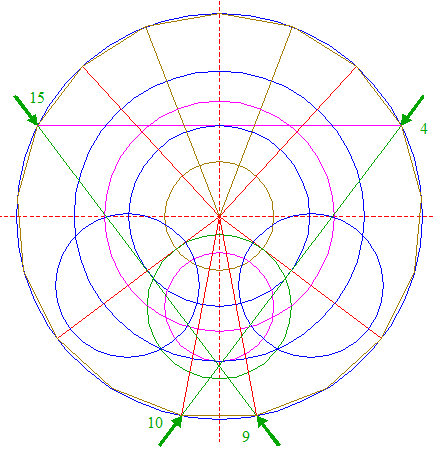Draw diagonals 4-10 and 9-15. 29.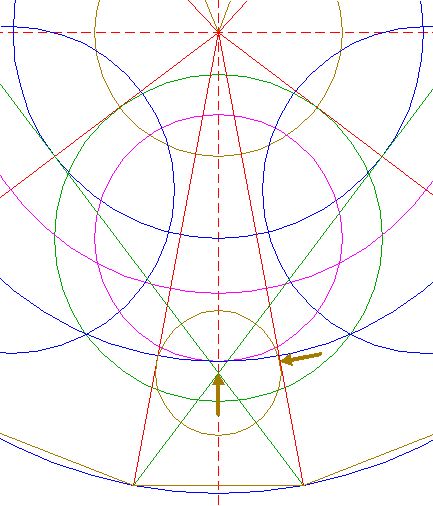Construct a circle centered at the intersection of lines 28, tangent to lines 27. 30.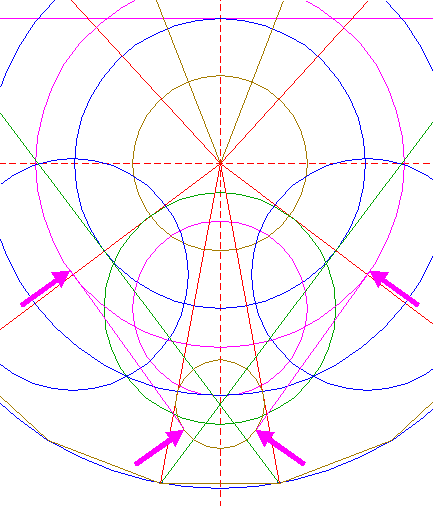Construct two lines tangent to circles 25 and 29 at the same (lower lefthand and lower righthand) sides. 31.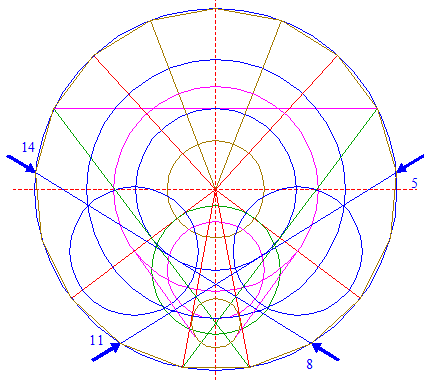Draw diagonals 5-11 and 8-14. 32.Construct two circles, both tangent to circle 29 at the upper sides, one tangent to the righthand circle 26 at the near side and tangent to diagonal 5-11, the other tangent to the lefthand circle 26 at the near side and tangent to diagonal 8-14, as shown. 33.Draw diagonals 6-12 and 7-13. 34.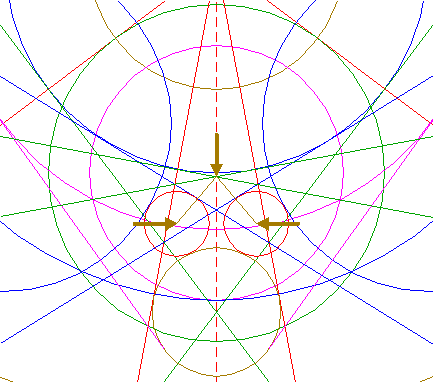Draw two lines, from the intersection of lines 33 to the centers of circles 32. 35.Construct two circles centered at the intersections of circles 32 and lines 34, tangent to the nearest line 27. 36.Draw the connecting line between the intersections of circles 18 and 29. 37.Construct a circle with line 36 as its centerline. 38.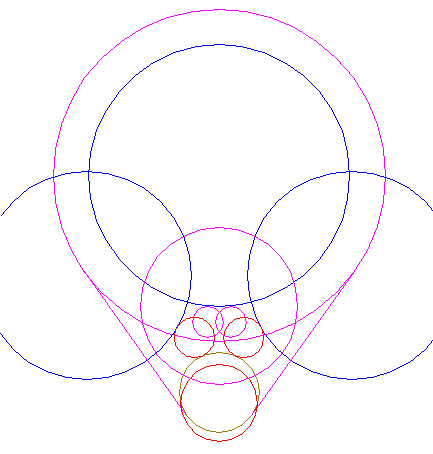Remove all redundant parts from circles 16, 20, 25, 26, 29, 32, 35, and 37, and lines 30,... 39....to create the shown pattern. 40.Construct a circle centered at the mid-point of the lower side of heptadecagon 14, tangent to circle 29 at the lower side. 41.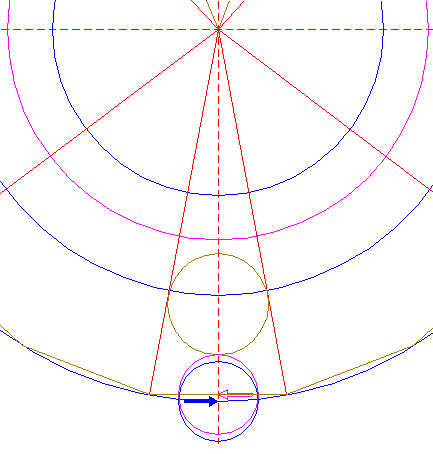Copy circle 40 to the lower intersection of circle 1 and the vertical centerline. 42.Construct a circle concentric to circle 1, tangent to circle 41 at the lower side. 43.Construct a "two-points" circle between the upper intersections of circles 16 and 25 with the vertical centerline. 44.Copy circle 43 to angular point 14 nr. 1. 45.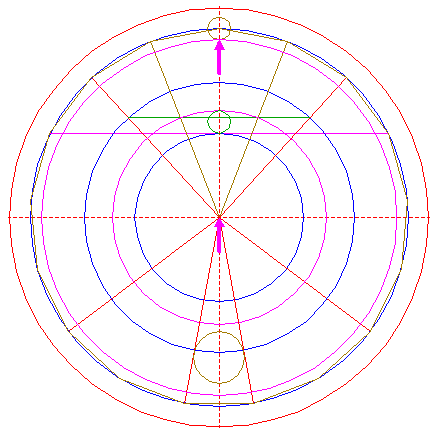Construct a circle concentric to circle 1, tangent to circle 44 at the lower side. 46.Construct a "two-points" circle between the upper intersection of circle 25, and the intersection of line 23 with the vertical centerline. 47.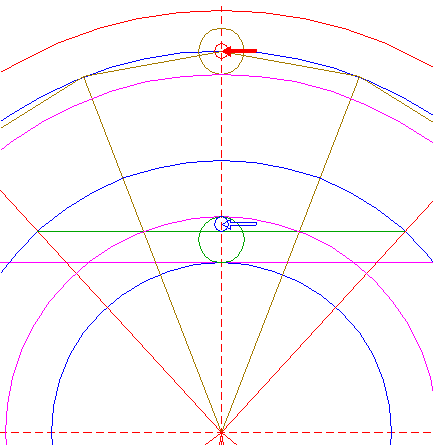Copy circle 46 to the center of circle 44. 48.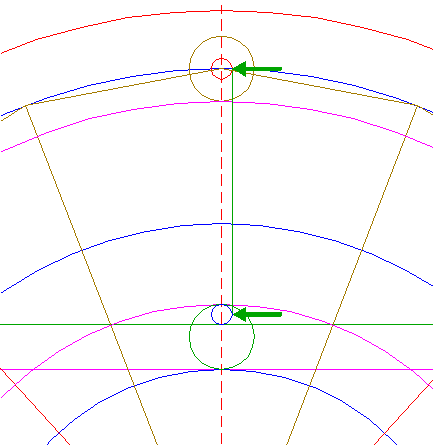Construct a line parallel to the vertical centerline, tangent to circles 46 and 47 at the righthand sides. 49.Copy circle 46 two times, to the intersections of circles 1 and 16 with ray 2. 50.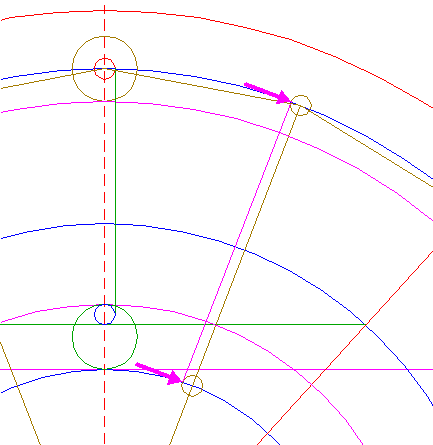Construct a line parallel to ray 2, tangent to circles 49 at the upper lefthand sides. 51.Construct a "two-points" circle between angular points 14 nrs. 1 and 2. 52.Draw a line between the centers of circles 1 and 51, and extend it upto circle 1. 53.Construct a "two-points" circle between the intersections of circle 1 and heptadecagon 14 with line 52. 54.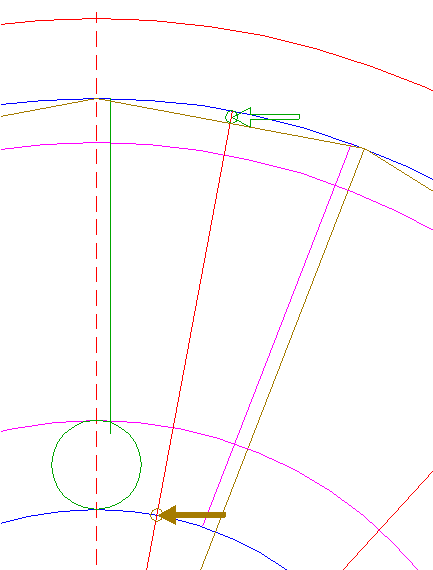Copy circle 53 to the intersection of circle 16 and line 52. 55.Construct two parallel lines, tangent to circles 53 and 54 at both sides. 56.Construct a circle enclosed by and tangent to circle 45, line 48 and lefthand line 55. 57.Copy circle 56, while mirroring with respect to line 52. 58.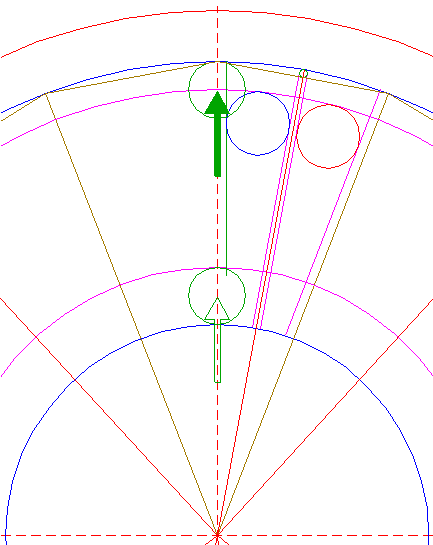Copy circle 43 to the upper intersection of circle 45 and the vertical centerline. 59.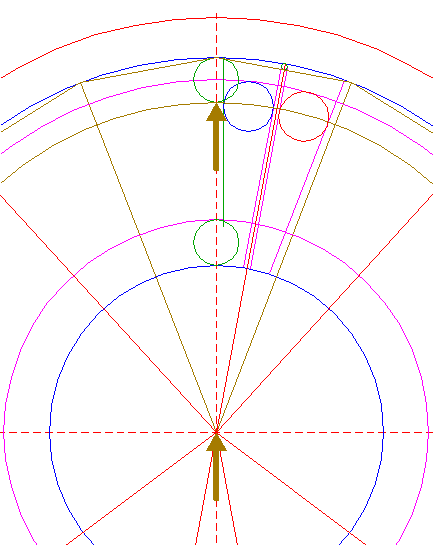Construct a circle concentric to circle 1, tangent to circle 58 at the lower side. 60.Construct a circle concentric to circle 56, tangent to the vertical centerline. 61.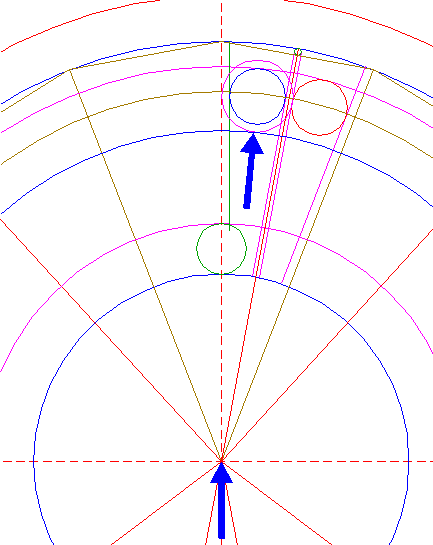Construct a circle concentric to circle 1, tangent to circle 60 at the lower side. 62.Construct a circle centered at the upper intersection of circle 61 and the vertical centerline, tangent to circle 59 at the upper side. 63.Construct a circle concentric to circle 1, tangent to circle 62 at the lower side. 64.Draw diagonals 2-13 and 6-17. 65.Copy circle 56 to the intersection of lines 64. 66.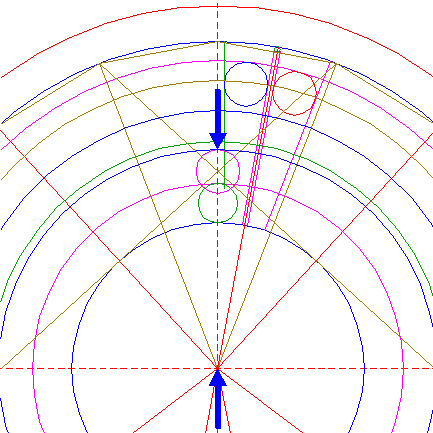Construct a circle concentric to circle 1, tangent to circle 65 at the upper side. 67.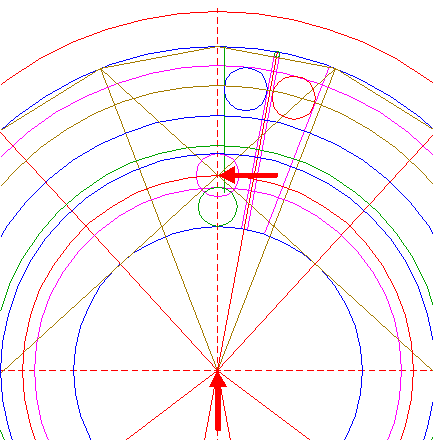Construct a circle concentric to circle 1, passing through the center of circle 65. 68.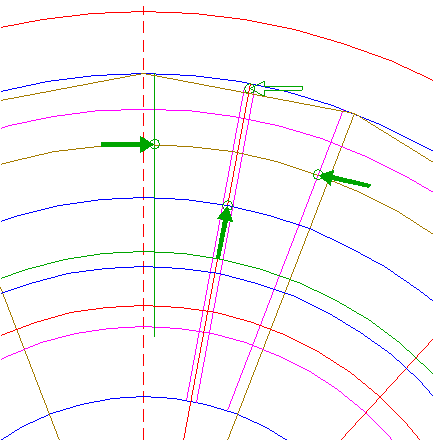Copy circle 53 three times, to the intersections of lines 48 and 50 with circle 59, and to the intersection of circle 61 and line 52. 69.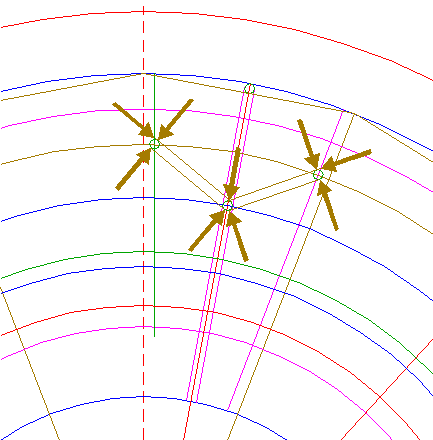Construct two pairs of parallel lines, both tangent to the lower circle 68, one tangent to the lefthand, and one tangent to the righthand circles 68, all at both sides, as shown. Extend the upper lines upto the nearest line 48 or 50. 70.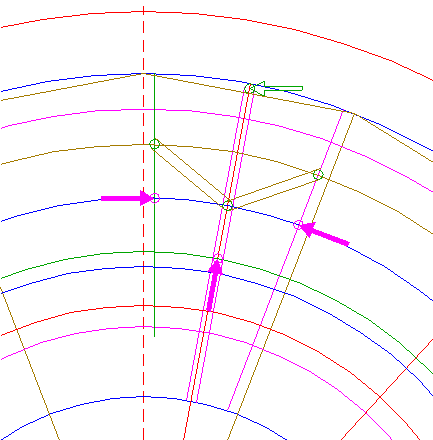Copy circle 53 three times, to the intersections of lines 48 and 50 with circle 61, and to the intersection of circle 63 and line 52. 71.Construct two pairs of parallel lines, similar to lines 69, with respect to circles 70. 72.Copy circle 53 three times, to the intersections of lines 48 and 50 with circle 66, and to the intersection of circle 67 and line 52. 73.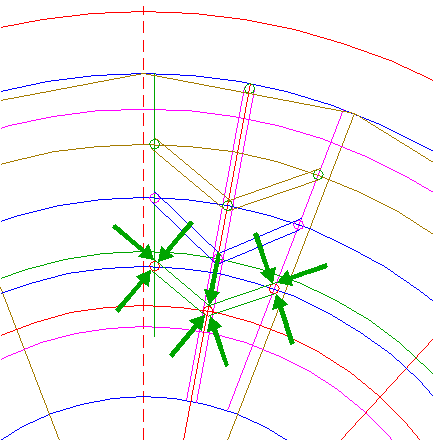Construct two pairs of parallel lines, similar to lines 69, with respect to circles 72. 74.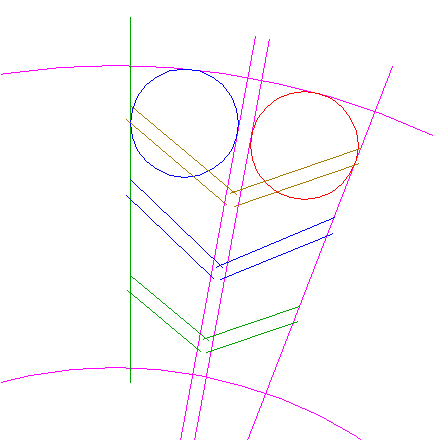Remove all redundant parts from circles 25, 45, 56, and 57, and from lines 48, 50, 55, 69, 71, and 73,... 75.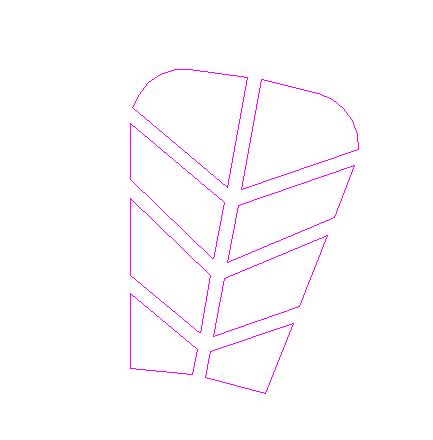...to create the shown pattern. 76.Copy pattern 75 thirteen times, from the mid-point of the upper righthand side of heptadecagon 14, to the corresponding points of the other sides, except for the lower three sides, while rotating around the center of circle 1. 77.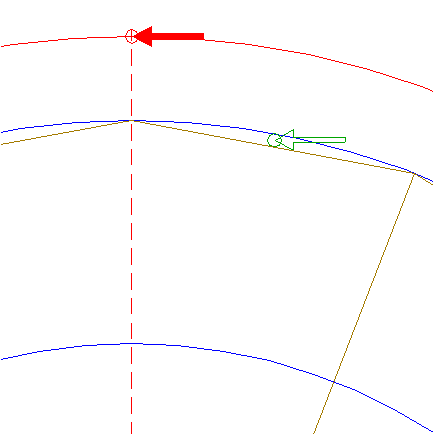Copy circle 53 to the upper intersection of circle 42 and the vertical centerline. 78.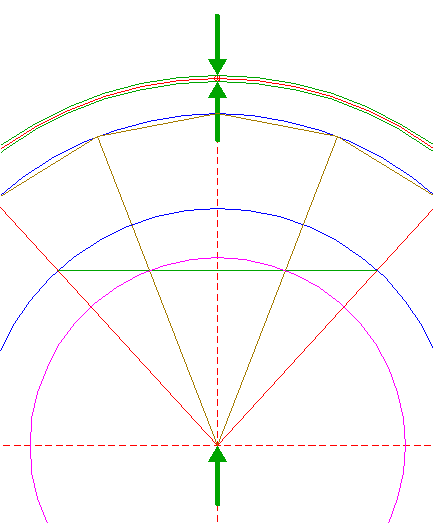Construct two circles concentric to circle 1, tangent to circle 77 at both sides. 79.Draw rays 8 and 11. 80.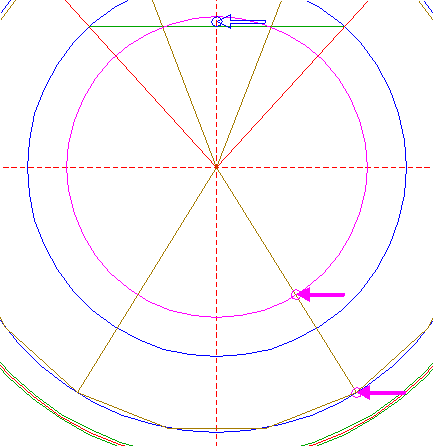Copy circle 46 two times, to the intersections of circles 1 and 25 with righthand line 79. 81.Construct a line parallel to righthand line 79, tangent to circles 80 at the lower lefthand sides. 82.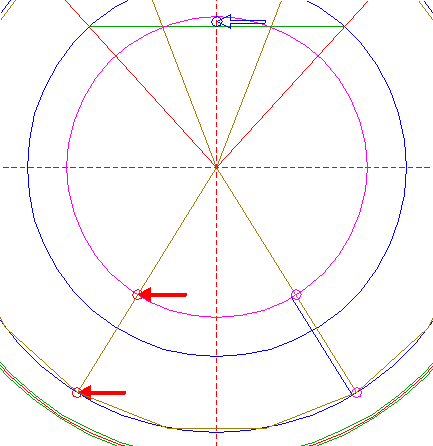Copy circle 46 two times, to the intersections of circles 1 and 25 with lefthand line 79. 83.Construct a line parallel to lefthand line 79, tangent to circles 82 at the lower righthand sides. 84.Circles 1, 45, and 78, lines 81, and 83, and patterns 39, 75, and 76, are used for the final reconstruction. 85.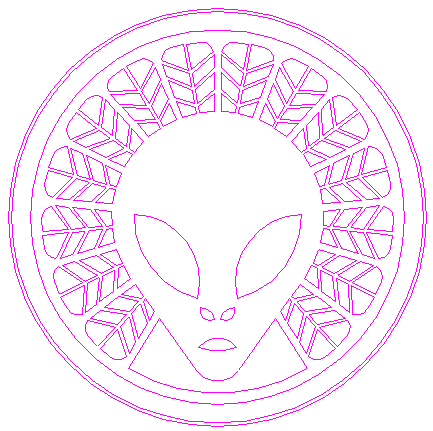Remove all parts not visible within the formation itself. 86.Colour all areas corresponding to standing... 87....or to flattened crop, and finish the reconstruction of the 2016 Reigate Hill formation. 88.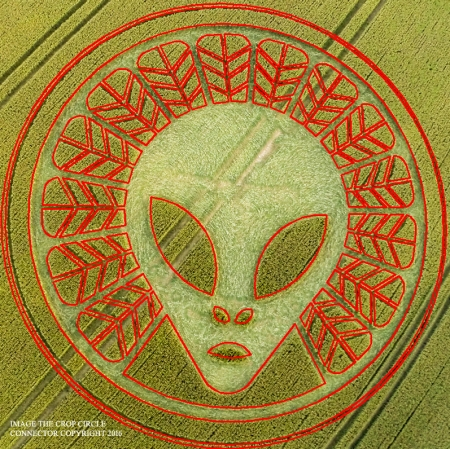The final result, matched with the aerial image.
 Copyright © 2016, Zef Damen, The Netherlands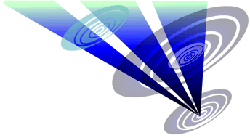Mark Fussell & Stuart Dike